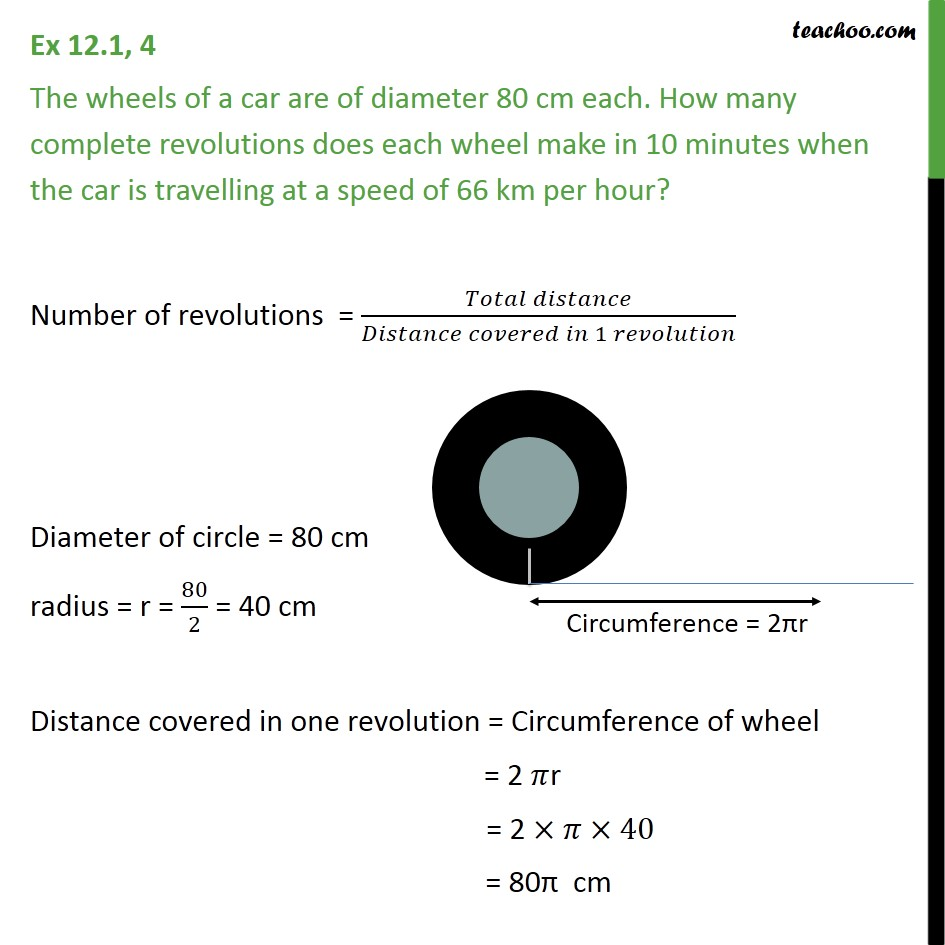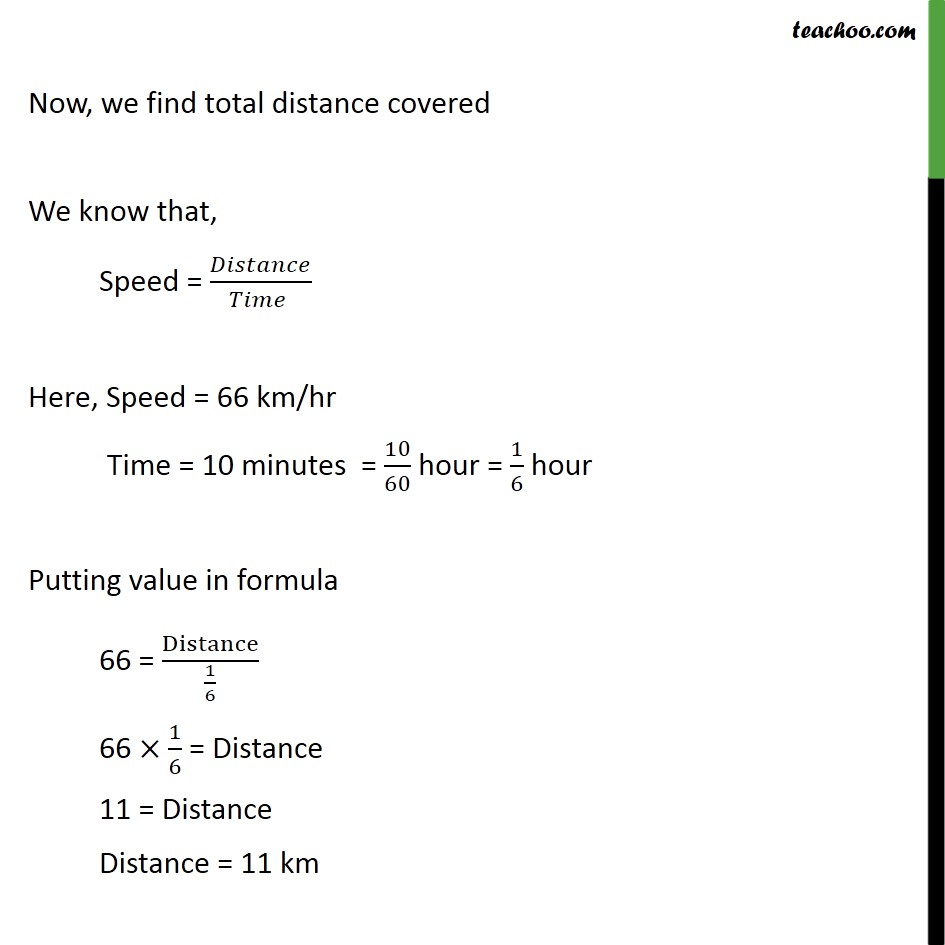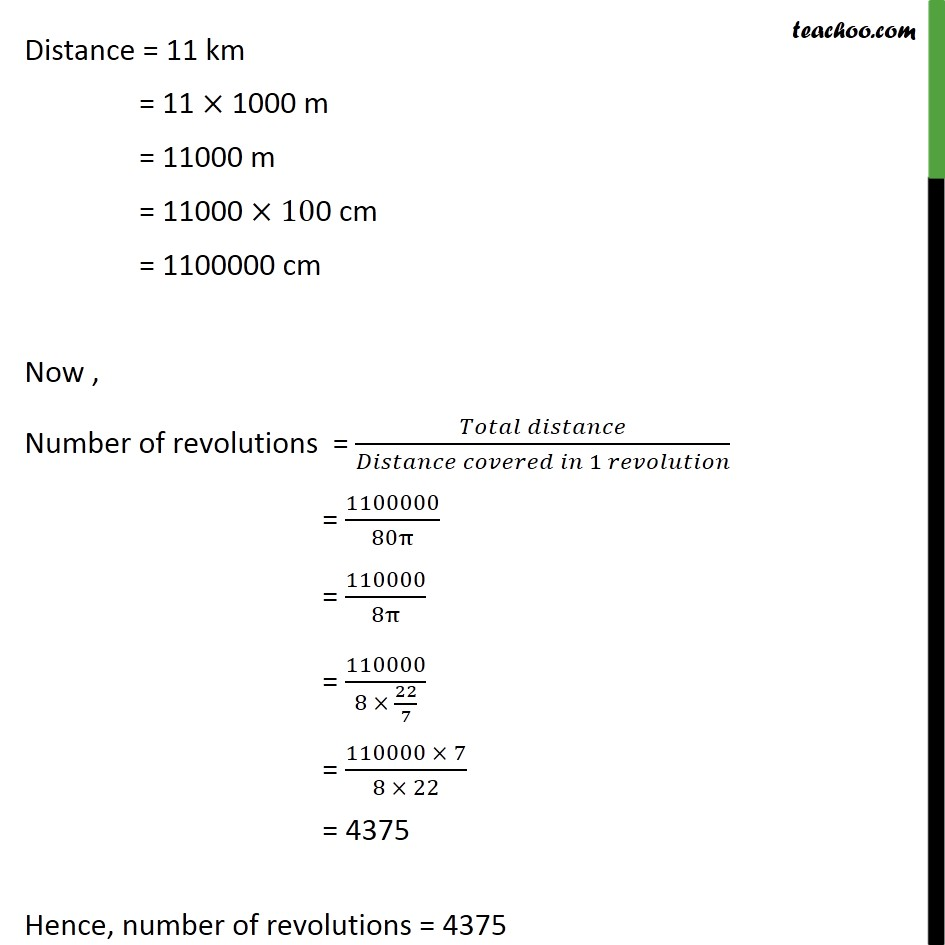1. Class 10
2. Important Questions for Exam - Class 10
3. Chapter 12 Class 10 Areas related to Circles

Transcript

Ex 12.1, 4 The wheels of a car are of diameter 80 cm each. How many complete revolutions does each wheel make in 10 minutes when the car is travelling at a speed of 66 km per hour? Number of revolutions = (𝑇𝑜𝑡𝑎𝑙 𝑑𝑖𝑠𝑡𝑎𝑛𝑐𝑒)/(𝐷𝑖𝑠𝑡𝑎𝑛𝑐𝑒 𝑐𝑜𝑣𝑒𝑟𝑒𝑑 𝑖𝑛 1 𝑟𝑒𝑣𝑜𝑙𝑢𝑡𝑖𝑜𝑛) Diameter of circle = 80 cm radius = r = 80/2 = 40 cm Distance covered in one revolution = Circumference of wheel = 2 𝜋r = 2 ×𝜋×40 = 80π cm Now, we find total distance covered We know that, Speed = 𝐷𝑖𝑠𝑡𝑎𝑛𝑐𝑒/(𝑇𝑖𝑚𝑒 ) Here, Speed = 66 km/hr Time = 10 minutes = 10/60 hour = 1/6 hour Putting value in formula 66 = Distance/(1/6) 66 ×1/6 = Distance 11 = Distance Distance = 11 km Distance = 11 km = 11 × 1000 m = 11000 m = 11000 ×100 cm = 1100000 cm Now , Number of revolutions = (𝑇𝑜𝑡𝑎𝑙 𝑑𝑖𝑠𝑡𝑎𝑛𝑐𝑒)/(𝐷𝑖𝑠𝑡𝑎𝑛𝑐𝑒 𝑐𝑜𝑣𝑒𝑟𝑒𝑑 𝑖𝑛 1 𝑟𝑒𝑣𝑜𝑙𝑢𝑡𝑖𝑜𝑛) = 1100000/80π = 110000/8π = 110000/(8 × 22/7) = (110000 × 7)/(8 × 22) = 4375 Hence, number of revolutions = 4375

Chapter 12 Class 10 Areas related to Circles

Class 10
Important Questions for Exam - Class 10